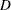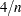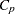ODS Graphics

Statistical procedures use ODS Graphics to create graphs as part of their output. ODS Graphics is described in detail in Chapter 21, Statistical Graphics Using ODS.

Before you create graphs, ODS Graphics must be enabled (for example, with the ODS GRAPHICS ON statement). For more information about enabling and disabling ODS Graphics, see the section Enabling and Disabling ODS Graphics in Chapter 21, Statistical Graphics Using ODS.

The overall appearance of graphs is controlled by ODS styles. Styles and other aspects of using ODS Graphics are discussed in the section A Primer on ODS Statistical Graphics in Chapter 21, Statistical Graphics Using ODS.

The following sections describe the ODS graphical displays produced by PROC REG.

### Diagnostics Panel

The "Diagnostics Panel" provides a display that you can use to get an overall assessment of your model. See Figure 76.8 for an example.

The panel contains the following plots:

• residuals versus the predicted values

• externally studentized residuals (RSTUDENT) versus the predicted values

• externally studentized residuals versus the leverage

• normal quantile-quantile plot (Q-Q plot) of the residuals

• dependent variable values versus the predicted values

• Cook’sversus observation number

• histogram of the residuals

• "Residual-Fit" (or RF) plot consisting of side-by-side quantile plots of the centered fit and the residuals

• box plot of the residuals if you specify the STATS=NONE suboption

Patterns in the plots of residuals or studentized residuals versus the predicted values, or spread of the residuals being greater than the spread of the centered fit in the RF plot, are indications of an inadequate model. Patterns in the spread about the 45-degree reference line in the plot of the dependent variable values versus the predicted values are also indications of an inadequate model.

The Q-Q plot, residual histogram, and box plot of the residuals are useful for diagnosing violations of the normality and homoscedasticity assumptions. If the data in a Q-Q plot come from a normal distribution, the points will cluster tightly around the reference line. A normal density is overlaid on the residual histogram to help in detecting departures form normality.

Following Rawlings (1998), reference lines are shown on the relevant plots to identify observations deemed outliers or influential. Observations whose externally studentized residual magnitudes exceed 2 are deemed outliers. Observations whose leverage value exceedsor whose Cook’svalue exceedsare deemed influential (is the number of regressors including the intercept, andis the number of observations used in the analysis). If you specify the LABEL suboption of the PLOTS=DIAGNOSTICS option, then the points deemed outliers or influential are labeled on the appropriate plots.

Fit statistics are shown in the lower right of the plot and can be customized or suppressed by using the STATS= suboption of the PLOTS=DIAGNOSTICS option.

### Residuals by Regressor Plots

Panels of plots of the residuals versus each of the regressors in the model are produced by default. Patterns in these plots are indications of an inadequate model. To help in detecting patterns, you can use the SMOOTH= suboption of the PLOTS=RESIDUALS option to add loess fits to these residual plots. See Figure 76.1.6 for an example.

### Fit and Prediction Plots

A fit plot consisting of a scatter plot of the data overlaid with the regression line, as well as confidence and prediction limits, is produced for models depending on a single regressor. Fit statistics are shown to the right of the plot and can be customized or suppressed by using the STATS= suboption of the PLOTS=FIT option.

When a model contains more than one regressor, a fit plot is not appropriate. However, if all the regressors in the model are transformations of a single variable in the input data set, then you can request a scatter plot of the dependent variable overlaid with a fit line and confidence and prediction limits versus this variable. You can also plot residuals versus this variable. You request these plots, shown in a panel, with the PLOTS=PREDICTION option. See Figure 76.13 for an example.

### Influence Plots

In addition to the "Cook’s D Plot" and the "RStudent By Leverage Plot," you can request plots of the DFBETAS and DFFITS statistics versus observation number by using the PLOTS=DFBETAS and PLOTS=DFFITS options. You can also obtain partial regression leverage plots by using the PLOTS=PARTIAL option. See the section Influence Statistics for examples of these plots and details about their interpretation.

### Ridge and VIF Plots

When you use ridge regression, you can request plots of the variance inflation factor (VIF) values and standardized ridge estimates by ridge values for each coefficient with the PLOTS=RIDGE option. See Example 76.5 for examples.

### Variable Selection Plots

When you request variable selection by using the SELECTION= option in the MODEL statement, you can request plots of fit criteria for the models examined by using the PLOTS=CRITERIA option. The fit criteria are displayed versus the step number for the FORWARD, BACKWARD, and STEPWISE selection methods and the step at which the optimal value of each criterion is obtained is indicated using a "Star" marker. For the all-subset-based selection methods (SELECTION=RSQUARE|ADJRSQ|CP), the fit criteria are displayed versus the number of observations in the model.

The criteria are shown in a panel, but you can use the UNPACK suboption of the PLOTS=CRITERIA option to obtain separate plots for each criterion. You can also use the LABEL suboption of the PLOTS=CRITERIA option to request that optimal models be labeled on the plots. Example 76.2 provides several examples.

### ODS Graph Names

PROC REG assigns a name to each graph it creates using ODS. You can use these names to reference the graphs when using ODS. The names are listed in Table 76.11.

Table 76.11 ODS Graphical Displays Produced by PROC REG

ODS Graph Name

Plot Description

PLOTS Option

AdjrsqPlot

Adjusted R-square statistic for models examined doing variable selection

ADJRSQ

AICPlot

AIC statistic for models examined doing variable selection

AIC

BICPlot

BIC statistic for models examined doing variable selection

BIC

CooksDPlot

Cook’sstatistic versus observation number

COOKSD

CPPlotstatistic for models examined doing variable selection

CP

DFFITSPlot

DFFITS statistics versus observation number

DFFITS

DFBETASPanel

Panel of DFBETAS statistics versus observation number

DFBETAS

DFBETASPlot

DFBETAS statistics versus observation number

DFBETAS(UNPACK)

DiagnosticsPanel

Panel of fit diagnostics

DIAGNOSTICS

FitPlot

Regression line, confidence limits, and prediction limits overlaid on scatter plot of data

FIT

ObservedByPredicted

Dependent variable versus predicted values

OBSERVEDBYPREDICTED

PartialPlot

Partial regression plot

PARTIAL

PredictionPanel

Panel of residuals and fit versus specified variable

PREDICTIONS

PredictionPlot

Regression line, confidence limits, and prediction limits versus specified variable

PREDICTIONS(UNPACK)

PredictionResidualPlot

Residuals versus specified variable

PREDICTIONS(UNPACK)

QQPlot

Normal quantile plot of residuals

QQ

ResidualBoxPlot

Box plot of residuals

BOXPLOT

ResidualByPredicted

Residuals versus predicted values

RESIDUALBYPREDICTED

ResidualHistogram

Histogram of fit residuals

RESIDUALHISTOGRAM

ResidualPlot

Plot of residuals versus regressor

RESIDUALS

RFPlot

Side-by-side plots of quantiles of centered fit and residuals

RF

RidgePanel

Plot of VIF and ridge traces

RIDGE

RidgePlot

Plot of ridge traces

RIDGE(UNPACK)

RSquarePlot

R-square statistic for models examined doing variable selection

RSQUARE

RStudentByLeverage

Studentized residuals versus leverage

RSTUDENTBYLEVERAGE

RStudentByPredicted

Studentized residuals versus predicted values

RSTUDENTBYPREDICTED

SBCPlot

SBC statistic for models examined doing variable selection

SBC

SelectionCriterionPanel

Panel of fit statistics for models examined doing variable selection

CRITERIA

VIFPlot

Plot of VIF traces

RIDGE(UNPACK)